# Atomic Theory and Chemical Symbols

## Chapter 3 - Chemistry in Industrial Instrumentation

The three “elementary” particles of matter comprising all atoms are electrons, protons, and neutrons. Combinations of these three particle types in various whole-number quantities constitute every type of atom. These fundamental particles are absolutely miniscule in comparison to the macroscopic existence of human beings. Just to illustrate, the mass of a single proton is approximately $$1.67 \times 10^{-27}$$ kilograms: written without scientific notation, it would be 0.00000000000000000000000000167 kg. An electron is even smaller: weighing in at $$9.11 \times 10^{-31}$$ kg (about 1800 times less mass than a proton!). Being far smaller in size than a wavelength of visible light, we cannot see these particles even with the most powerful optical microscope.

Protons and neutrons are very tightly bound together in the nucleus (center) of an atom. This bond is so secure that only extraordinary forces are able to pry an atom’s nucleus apart. Suffice it to say, one cannot disturb the stability of an atomic nucleus by rubbing, cutting, grinding, heating, smashing, or any other macroscopic physical process. The force binding protons and neutrons together in the nucleus is known as the strong nuclear force.

Electrons “orbit” the nucleus of atoms, and are held in proximity to those nuclei by electrostatic attraction (the so-called electromagnetic force), which is many orders of magnitude weaker than the strong nuclear force. Thus, electrons can be dislodged from or added to atoms through the action of macroscopic forces such as rubbing, cutting, grinding, heating, etc. It is the changeable configurations of electrons that accounts for different atoms joining together to form molecules.

The chemical identity of any atom is a simple and direct function of how many protons that atom has in its nucleus. Each nitrogen atom, for example, has seven (7) protons in its nucleus. This quantity is called the atomic number of an atom. In order for an atom to have a net neutral electric charge, there must be as many electrons orbiting the nucleus as there are protons in the nucleus, since protons are positively charged and electrons are negatively charged (equal and opposite electric charges, each). Therefore, a neutral atom of nitrogen will have seven electrons orbiting around the nucleus, electrically balancing the seven protons within the nucleus.

The number of neutrons within the nucleus of an atom does not affect the atom’s chemical identity, but it may affect its nuclear properties (e.g. whether or not it is radioactive; to what degree it captures certain forms of radiation, etc.). For example, most nitrogen atoms have seven neutrons along with seven protons in their nuclei, giving a total nuclear particle count of fourteen – the atomic mass of the atom, sometimes called the atomic weight. However, it is possible for a nitrogen atom to have eight neutrons (an atomic mass of fifteen) and still be “nitrogen,” with all the same chemical properties.

A tremendously simplified model of a common nitrogen atom is shown here, with 7 protons and 7 neutrons in the nucleus, and 7 electrons in “orbit” around the nucleus: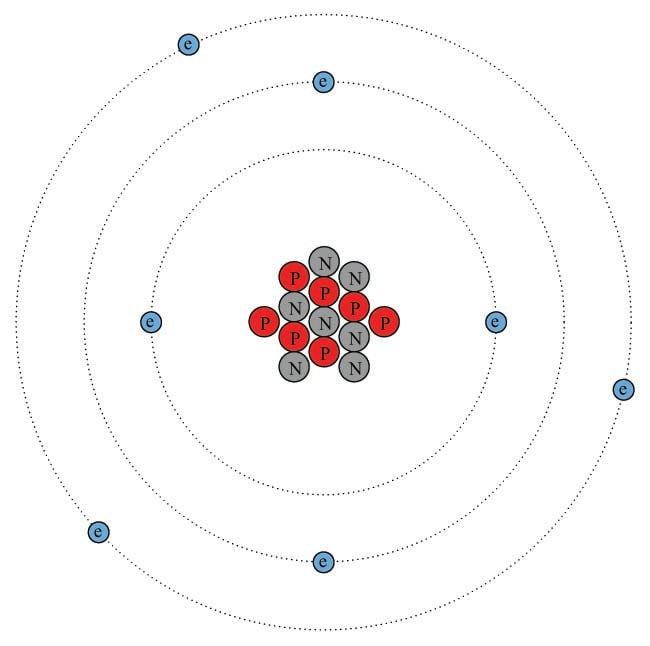The atomic number of this atom (the number of protons in the nucleus) is seven, which is what defines it as nitrogen. The atomic mass of this atom (the sum of protons and neutrons in the nucleus) is fourteen. The chemical symbol for this atom is shown here: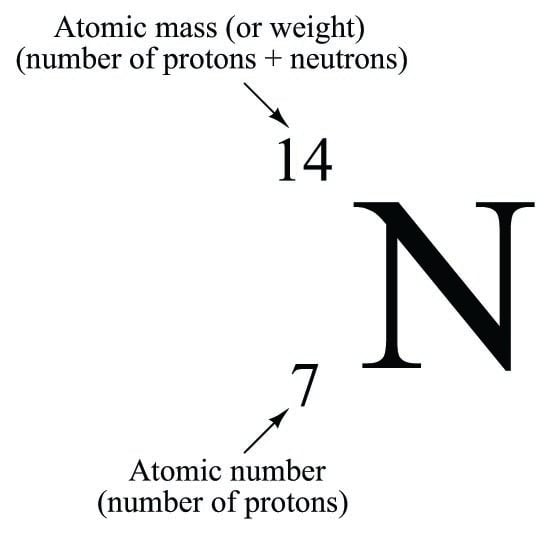The atomic number is redundant to the letter “N” for nitrogen, since only the element nitrogen can have an atomic number of seven. The atomic mass is only relevant when we need to distinguish one isotope of nitrogen from another (variations of elements having the same number of protons but different numbers of neutrons), and this is seldom because the chemical properties of isotopes are identical – only their masses differ. For these reasons, you will usually find no left-hand subscripts or superscripts placed near chemical symbols of elements in chemical expressions.

By contrast, subscripts and superscripts placed to the right of a chemical symbol have very important meanings in chemistry. A right-hand subscript refers to the number of atoms bound together to form a molecule. A right-hand superscript refers to the electrical charge possessed by an atom (or by a molecule) by virtue of the number of electrons not matching the number of protons:

An N$$_{2}$$ molecule may be represented simplistically as follows, the two nitrogen atoms joined by a mutual sharing of three of its highest-energy (valence) electrons, shown in this illustration as those electrons residing in the largest-diameter “orbits”. Incidentally, this “triple bond” characterizing the nitrogen molecule is very strong (the more electrons participating in the joining of two atoms, the stronger the bond, all other factors being equal):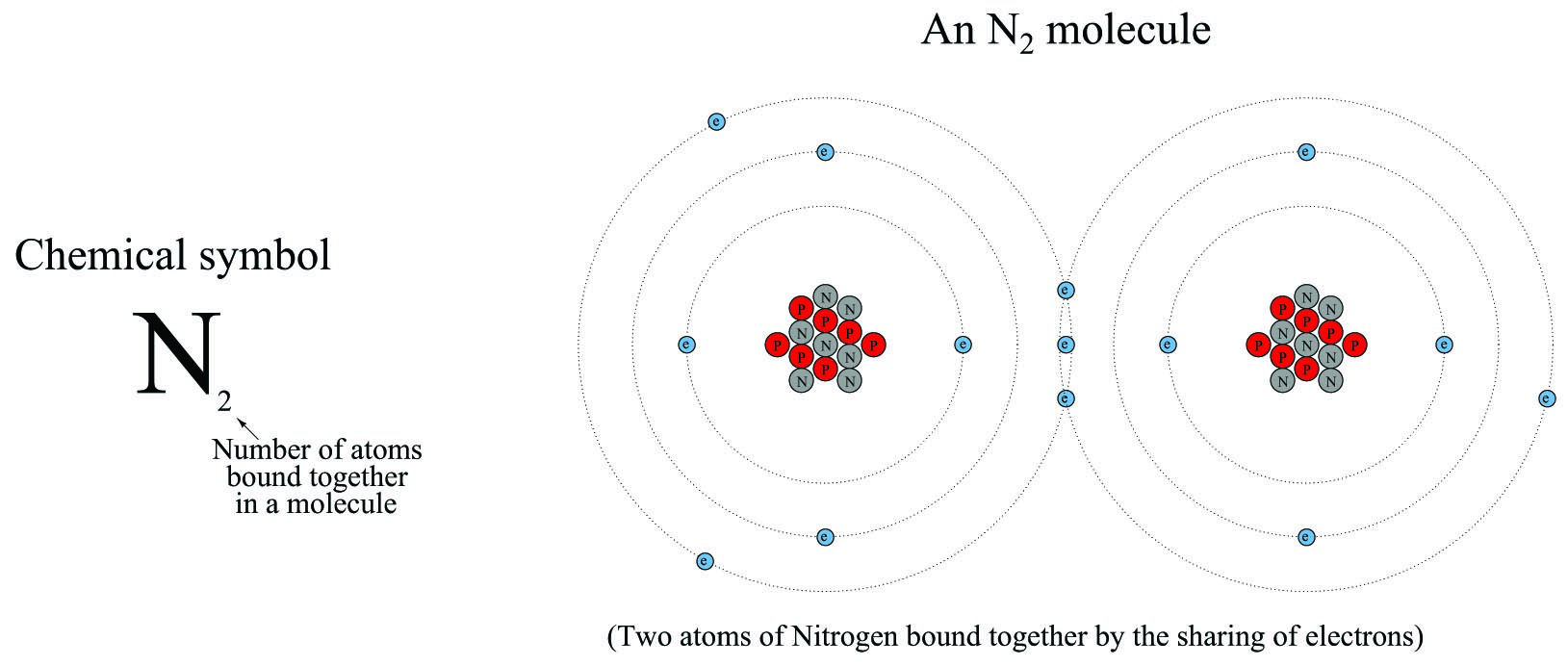An N$$^{3-}$$ ion is an atom of nitrogen having three more electrons than it normally would when electrically balanced: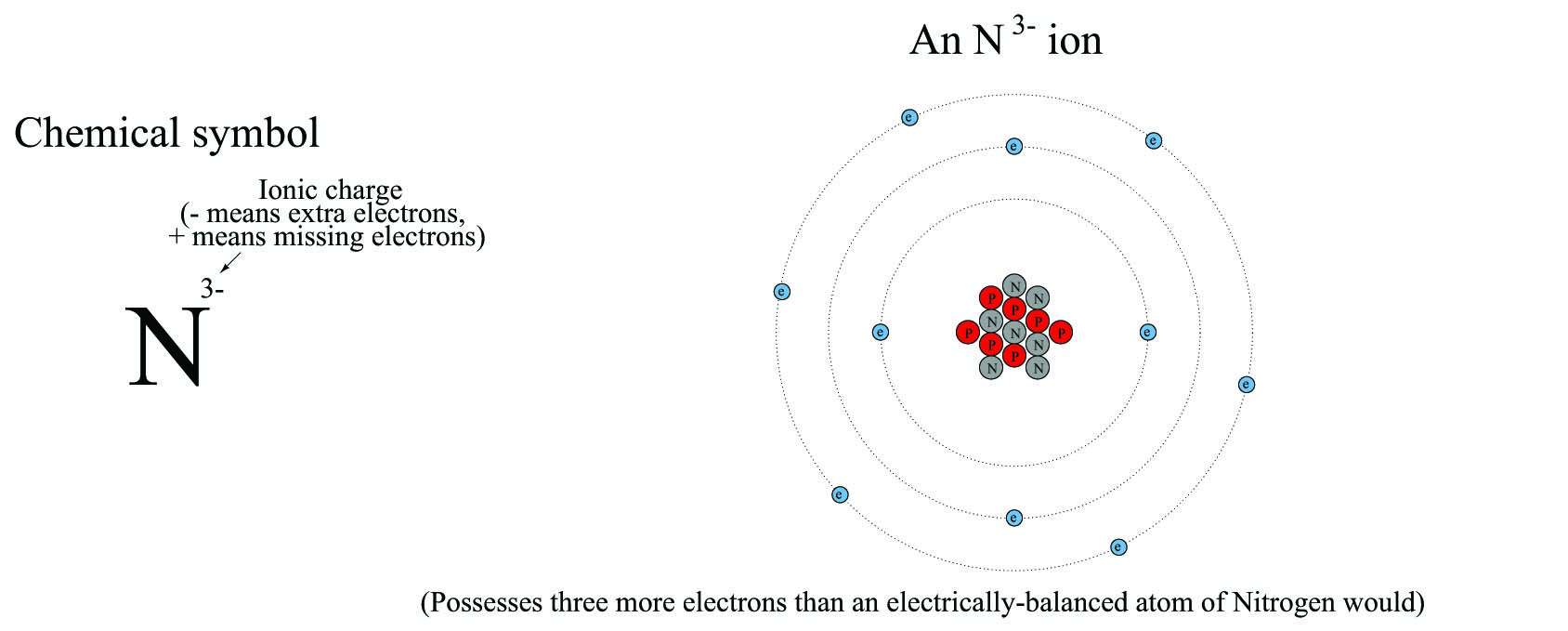A chemical formula is a written description of a molecule’s constituent atoms. Ethanol (ethyl alcohol), for example, is a conglomerate of two carbon atoms, six hydrogen atoms, and one oxygen atom. One way to express this structure is to write the following formula for ethanol, the right-hand subscripts showing the relative quantities of atoms in each ethanol molecule:

$\hbox{C}_2 \hbox{H}_6 \hbox{O}$

This is called a molecular formula, because it shows the proportions of atom types comprising each molecule.

A more common way to write the formula for ethanol, though, is this:

$\hbox{C}_2 \hbox{H}_5 \hbox{OH}$

Here, an attempt is made to show the physical structure of the ethanol molecule, where one of the hydrogen atoms is located further away from the others. This is called a structural formula. If more detail of the bonds between atoms in a molecule is needed, a semi-graphic representation called a displayed formula (also known as an expanded structural formula) may be used in lieu of a structural formula: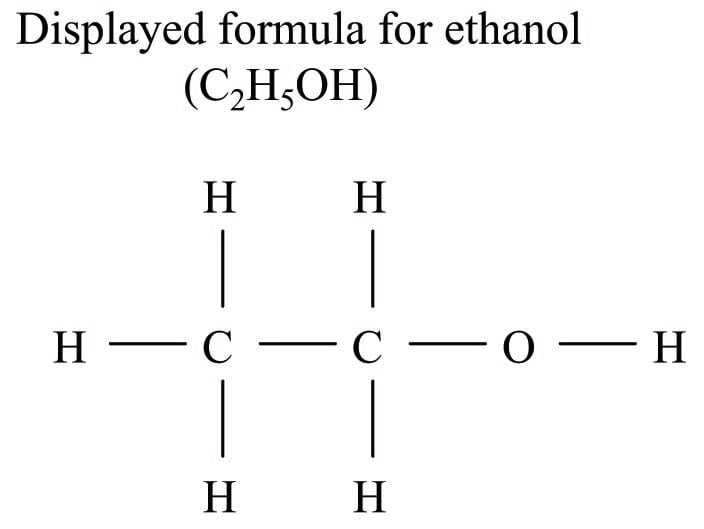Each letter in a displayed formula represents a single atom within that molecule, and each line segment in a displayed formula represents a bond between two.

In organic chemistry – the study of molecules principally centered around carbon atoms – a special type of notation is used to show the structural detail of the molecule with fewer lines and letters than a displayed formula. This notation is called a line drawing, where each line segment represents a single electron bond to a carbon atom, each vertex and line-end represents the location of a carbon atom, and any hydrogen atoms directly bound to a carbon atom are simply omitted for simplicity. Compare and contrast these displayed formulae and line drawings for a few different organic compounds: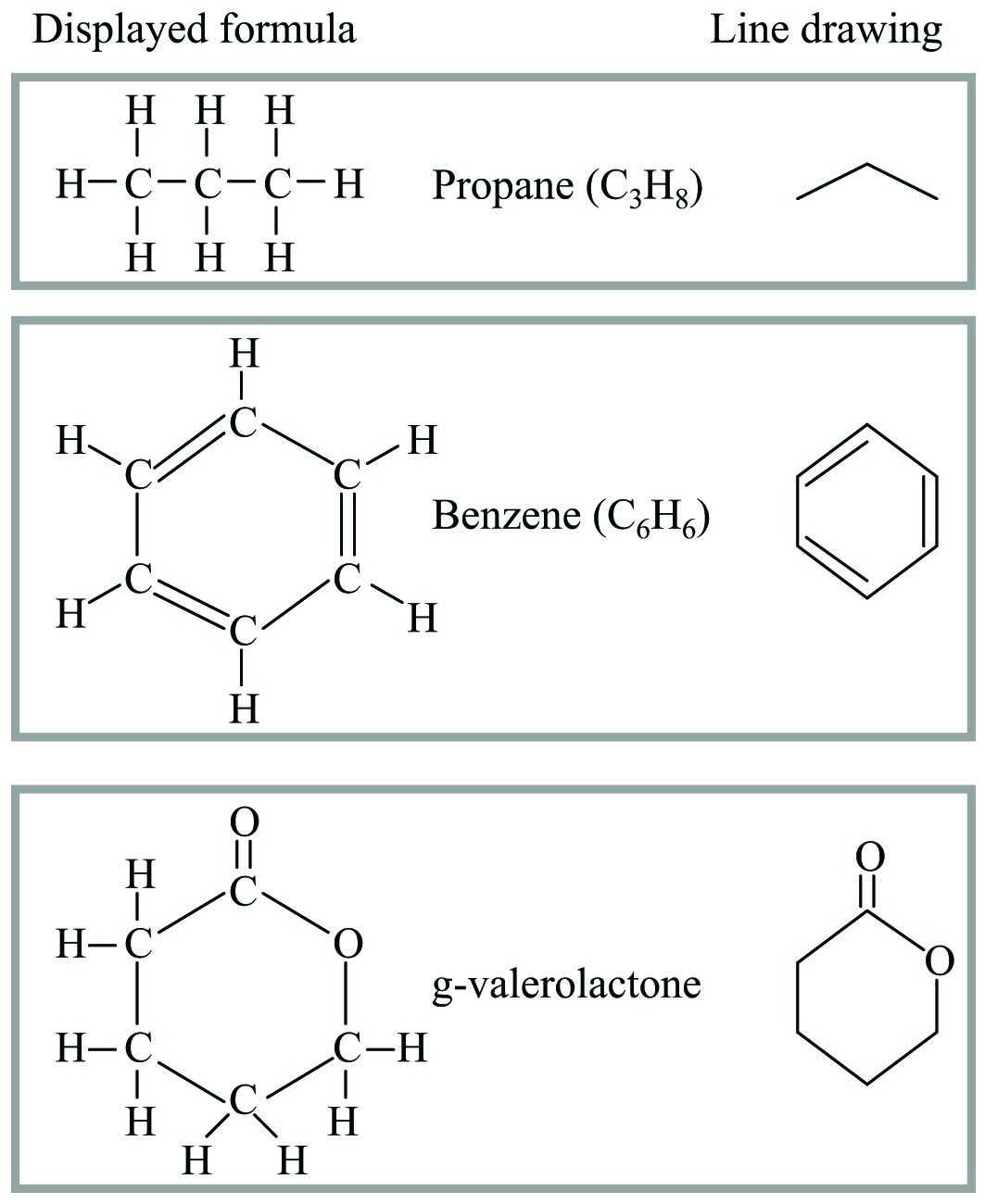An important principle in organic chemistry is that carbon atoms prefer to form exactly four bonds with surrounding atoms. This fact is exploited in line-drawing notation where any bonds not explicitly shown at a vertex are assumed to be single-bonds with hydrogen atoms, enough of them to bring the total number of bonds with that carbon atom to four. Since a great many organic compounds are principally comprised of carbon and hydrogen, the line-drawing symbols for these molecules tend to be more lines than letters.

Chemical engineers often perform mass and energy balance calculations for processes where mixtures of similar compounds exist. Wastewater treatment is one example, where an array of organic compounds must all be treated through oxidation (chemical reaction with oxygen). In such cases, it is common for chemical engineers to write formulae expressing the average ratios of elements, so that they may calculate the quantity of reactant(s) needed to complete the desired chemical reaction with compounds in the mixture. Primary sludge clarified from municipal wastewater, for example, may be represented by the compositional formula C$$_{22}$$H$$_{39}$$O$$_{10}$$N. This does not suggest the existence of some monstrous molecule consisting of twenty-two carbon atoms, thirty-nine hydrogen atoms, ten oxygen atoms, and a lone nitrogen atom somewhere in a sample of sludge, but rather that the combined average carbon, hydrogen, oxygen, and nitrogen quantities in that sludge exist in a variety of molecular forms in these approximate proportions. This aggregate formula expression helps the engineer quantify the gross chemical characteristics of the sludge, and from that determine how much oxygen will be necessary to completely oxidize it.

Sometimes, compositional formulae are written with non-integer subscripts. An example of this would be the compositional formula C$$_{4.8}$$H$$_{8.4}$$O$$_{2.2}$$, which also happens to be an average composition for municipal wastewater sludge (ignoring nitrogen content). The same formula could just as well have been written C$$_{48}$$H$$_{84}$$O$$_{22}$$, or even C$$_{24}$$H$$_{42}$$O$$_{11}$$, because these subscript values all express the exact same proportions.

Published under the terms and conditions of the Creative Commons Attribution 4.0 International Public License